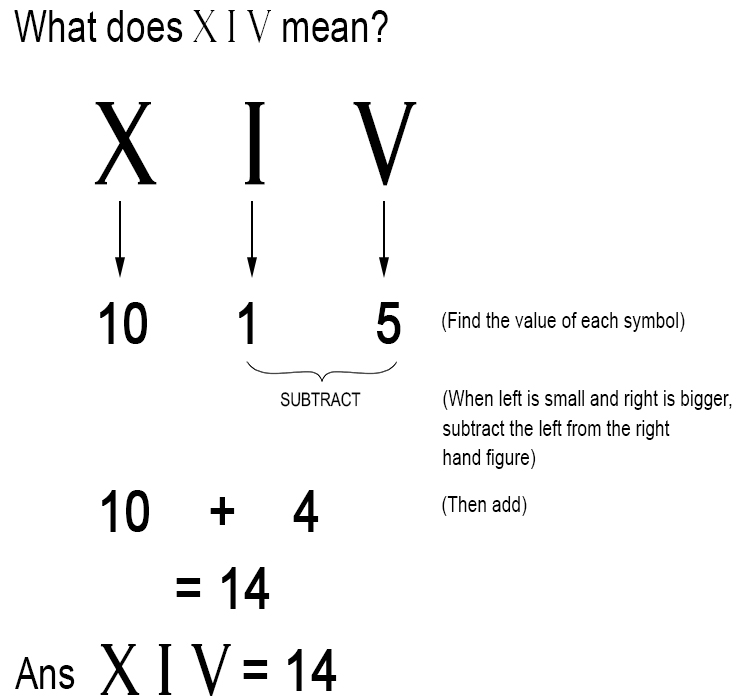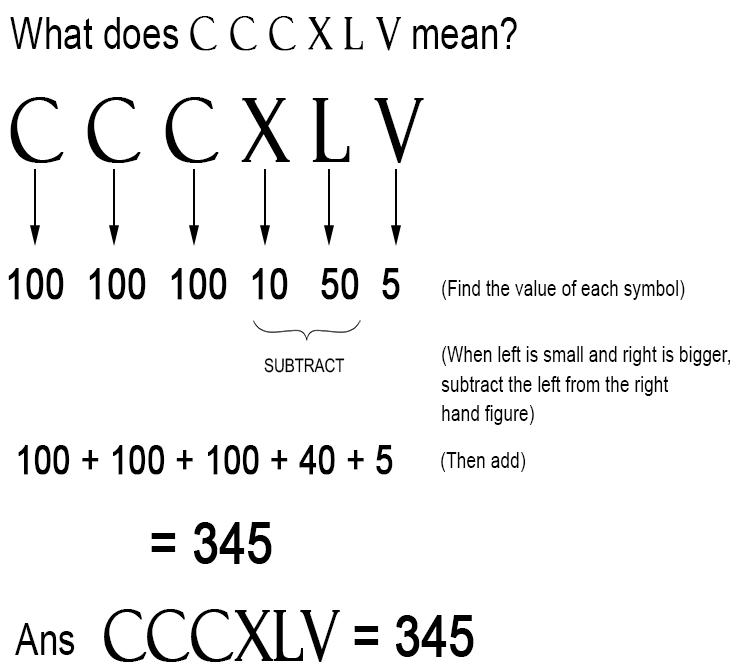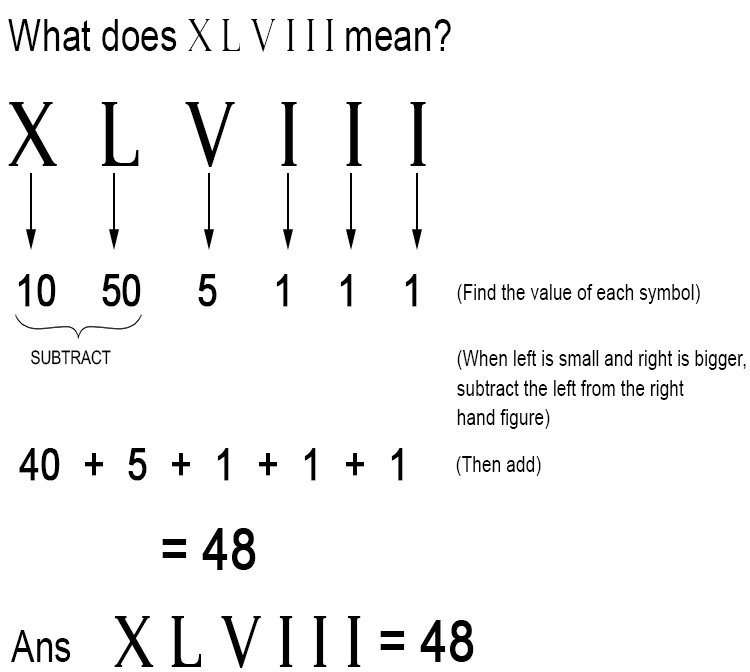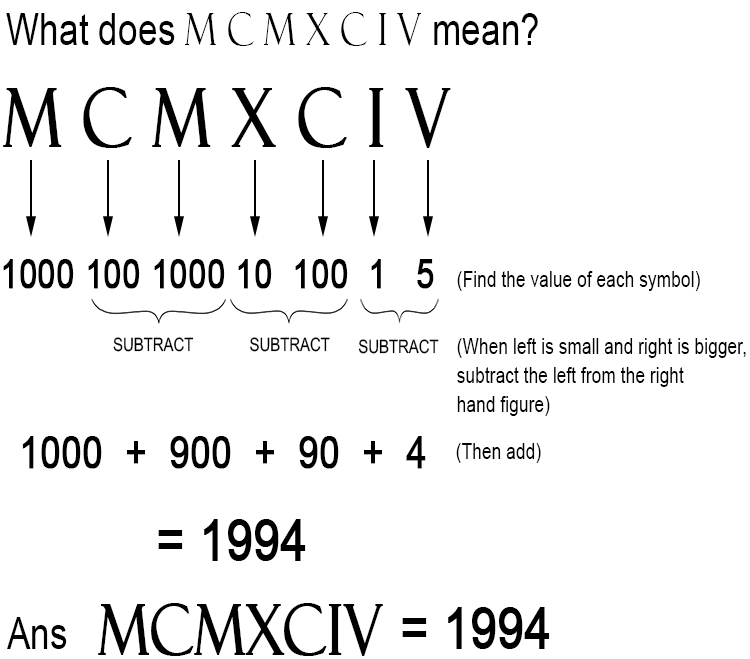# How to convert Roman numerals into numbers

Find the value of each symbol.
First subtract.

Subtract because as you see in Roman Numerals Rules:
When 'left' is smaller and 'right' is bigger, subtract the left from the right-hand figure.

Remember :
'I Value Xylophones Like Cows Do Milk'.
I=1,  V=5,  X=10,  L=50,  C=100,  D=500,  M=1000

Example 1Example 2Example 3Example 4